## 7.1The k-epsilon turbulence model

The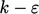model (k-epsilon) was the ﬁrst turbulence model to be widely adopted for a variety of ﬂows in CFD.2 It is one of a family of two equation models which solve two transport equations, one usually for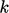and one for another variable, often a dissipation rate. These models are the industry standard for CFD.

Themodel combines the transport equations forand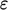, Eq. (6.28 ) and Eq. (6.32 ) respectively, which are reproduced below assuming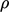= constant and replacing material derivatives in conservative form as in Eq. (2.26 ).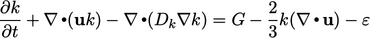(7.1)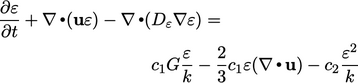(7.2)

The standard model coeﬃcients are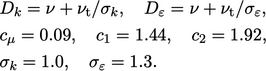(7.3)
The choice of coeﬃcients in Eq. (7.3 ) originates from a large series of validation simulations of free shear ﬂows.3

The model can be deployed in a steady ﬂow solution, setting the local time derivatives to zero, e.g.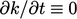. It can also form part of a transient solution to capture unsteady features such as vortex shedding.

The model uses a momentum equation in ensemble-averaged form, e.g. Eq. (6.26 ), using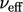calculated from updated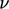and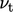according to Eq. (6.27 ).

The model equations are added to the end of the main loop in the steady and transient algorithms from Sec. 5.12 or Sec. 5.21 , respectively. The ﬁgure below shows the additions to the transient algorithm, starting from the momentum corrector step.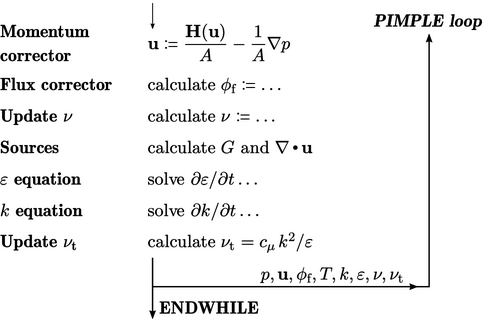Following the momentum and ﬂux correctors,can be updated, e.g. for non-Newtonian models where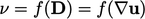.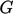and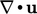are calculated and stored for source terms in both the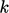and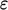equations. Those equations are then solved, followed by an update to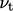, and subsequently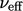.

2William Jones and Brian Launder, The prediction of laminarization with a two-equation model of turbulence, 1972.
3Brian Launder, Alan Morse, Wolfgang Rodi and Brian Spalding, Prediction of free shear ﬂows — A comparison of the performance of six turbulence models, NASA Conference on Free Shear Flows, Langley, 1972.

Notes on CFD: General Principles - 7.1 The k-epsilon turbulence model